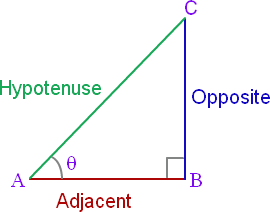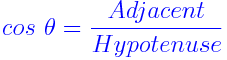# Cosine Formula

In trigonometry, the law of cosines is also known as the cosine formula or cosine rule, relates the lengths of the sides of a triangle to the cosine of one of its angles.## Formula for Cosine

The Cosine Formula is,

##• a2 b2 c2 − 2bc.cosA
• ba2 +c− 2ac.cosB
• cab− 2ab.cosC

### Solved Examples

Question 1: Calculate the cosine angle of a right triangle given the adjacent side and hypotenuse are 12 cm and 15 cm respectively ?
Solution:

Given,